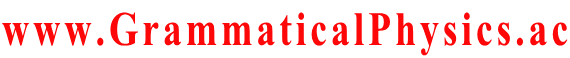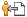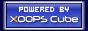Welcome SourceCodeOf_HumanGenome
www.GrammaticalPhysics.ac
Account
Access Detector
2 user(s) are online (1 user(s) are browsing Forum)

Members: 1
Guests: 1

SourceCodeOf_HumanGenome more...

# Correspondence between Solution and Action

SourceCodeOf_HumanGenome > Correspondence between Solution and Action @ 2013/4/15 14:42
A solution of the new grammar version of the Shrödinger equation:
Φ[χ]＝ΠkG(χ(k/α),k/α;x0,t0)
can be cartoonized as a picture of a starfish.

The integrand of the Feynman path integral:
exp{(i/h)S[χ]}＝ΠkG(χ(k/α + 1/α), k/α + 1/α; χ(k/α), k/α)
can be cartoonized as a picture of a centipede.

Using these two pictures as a hint, I made up the following functional as a solution nearly corresponding to the action.
Φ[χ]＝[Πk∫dxk∫dtkG(χ(k/α),k/α;xk,tk)]ΠjG(xj+1, tj+1; xj, tj)

This solution can be called a starfish having a centipede in place of a central disc.

k∫dξ(0,k/α) G(ξ(ε,k/α),ε;ξ(0,k/α),0)]Φ[ξ(0,□)]
= [Πk∫dξ(0,k/α) G(ξ(ε,k/α),ε;ξ(0,k/α),0)][Πs∫dxs∫dtsG(ξ(0,s/α),s/α;xs,ts)]ΠjG(xj+1, tj+1; xj, tj)
= [Πk∫dxk∫dtk∫dξ(0,k/α) G(ξ(ε,k/α),ε;ξ(0,k/α),0)G(ξ(0,k/α),k/α;xk,tk)]ΠjG(xj+1, tj+1; xj, tj)
= [Πk∫dxk∫dtk G(ξ(ε,k/α),k/α+ε;xk,tk)]ΠjG(xj+1, tj+1; xj, tj)
= [Πk'∫dx'k'∫dt'k' G(ξ(ε,k'/α-ε),k'/α;x'k',t'k')]ΠjG(x'j+1, t'j+1; x'j, t'j)　where k'=k+αε, x'k'=xk, t'k'=tk
= [Πk∫dx'k∫dt'k G(ξ(ε,k/α-ε),k/α;x'k,t'k)]ΠjG(x'j+1, t'j+1; x'j, t'j)
= [Πk∫dxk∫dtk G(ξ(ε,k/α-ε),k/α;xk,tk)]ΠjG(xj+1, tj+1; xj, tj)
= Φ[ξ(ε,□-ε)]
∵ Πj G(x'j+1, t'j+1; x'j, t'j)
= Πj' G(x'j'+1, t'j'+1; x'j', t'j')
= Πj' G(xj+1, tj+1; xj, tj)
= Πj G(xj+1, tj+1; xj, tj)
where j'=j+αε

Therefore,
Φ[χ]＝[Πk∫dxk∫dtkG(χ(k/α),k/α;xk,tk)]ΠjG(xj+1, tj+1; xj, tj)
is an approximate solution.

I hope that a path integral of this solution reproduce the same result as a path integral of exp{(i/h)S[χ]}.

---
Last edited at 2013/04/21/14:09

Category
Forum
Global

Notification method is private message [Change]

Site Search
Site Info
WebmasterswooderRecommend Us